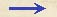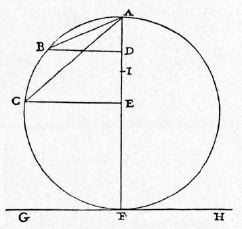Discorsi Propositions 2/06-th-06 Discorsi Proposition2/06-th-06THEOREMA VI, PROPOSITIO VI. THEOREM VI, PROPOSITION VISi a puncto sublimi vel imo circuli ad horizontem erecti ducantur quaelibet plana usque ad circumferentiam inclinata, tempora descensuum {10} per ipsa erunt aequalia. If from the highest or lowest point in a vertical circle there be drawn any inclined planes meeting the circumference the times of descent along these chords are each equal to the other. Sit circulus ad horizontem GH erectus, cuius ex imo puncto, nempe ex contactu cum horizontali, sit erecta diameter FA, et ex punto sublimi A plana quaelibet inclinentur usque ad circumferentiam AB, AC: dico, tempora descensuum per ipsa esse aequalia. Ducantur BD, CE ad diametrum perpendiculares, et inter planorum EA, AD altitudines media sit proportionalis AI: et quia rectangula FAE, FAD aequalia {20} sunt quadratis AC, AB; ut autem rectangulum FAE ad rectangulum FAD, ita EA ad AD; ergo ut quadratum CA ad quadratum AB, ita EA linea ad lineam AD. Verum ut linea EA ad DA, ita quadratum IA ad quadratum AD; ergo quadrata linearum CA, AB sunt inter se ut quadrata linearum IA, AD, et ideo ut CA linea ad AB, ita IA ad AD. At in praecedenti demonstratum est, rationem temporis descensus per AC ad tempus descensus per AB componi ex rationibus CA ad AB et DA ad AI, quae est eadem cum ratione BA ad AC; ergo ratio temporis descensus per AC ad {30} tempus descensus per AB componitur ex rationibus CA ad AB et BA ad AC; est igitur ratio eorumdem temporum ratio aequalitatis: ergo patet propositum. On the horizontal line GH construct a vertical circle. From its lowest point-the point of tangency with the horizontal-draw the diameter FA and from the highest point, A, draw inclined planes to B and C, any points whatever on the circumference; then the times of descent along these are equal. Draw BD and CE perpendicular to the diameter; make AI a mean proportional between the heights of the planes, AE and AD; and since the rectangles FA.AE and FA.AD are respectively equal to the squares of AC and AB, while the rectangle FA.AE is to the rectangle FA.AD as AE is to AD, it follows that the square of AC is to the square of AB as the length AE is to the length AD. But since the length AE is to AD as the square of AI is to the square of AD, it follows that the squares on the lines AC and AB are to each other as the squares on the lines AI and AD, and hence also the length AC is to the length AB as AI is to AD. (Condition 2/05-th-05) But it has previously been demonstrated that the ratio of the time of descent along AC to that along AB is equal to the product of the two ratios AC to AB and AD to AI; but this last ratio is the same as that of AB to AC. Therefore the ratio of the time of descent along AC to that along AB is the product of the two ratios, AC to AB and AB to AC. The ratio of these times is therefore unity. Hence follows our proposition.Idem aliter demonstratur ex mechanicis: nempe, in sequenti figura, mobile temporibus aequalibus pertransire CA, DA. By use of the principles of mechanics [ex mechanicis] one may obtain the same result, namely, that a falling body will require equal times to traverse the distances CA and DA, indicated in the following figure. {222} Sit enim BA aequalis ipsi DA, et ducantur perpendiculares BE, DF: constat ex elementis mechanicis, momentum ponderis super plano secundum lineam ABC elevato ad momentum suum totale esse ut BE ad BA, eiusdemque ponderis momentum super elevatione AD ad totale suum momentum esse ut DF ad DA vel BA; ergo eiusdem ponderis momentum super plano secundum DA inclinato ad momentum super inclinatione {10} secundum ABC est ut linea DF ad lineam BE; quare spatia, quae pertransibit idem pondus temporibus aequalibus super inclinationibus CA, DA, erunt inter se ut lineae BE, DF, ex propositione secunda primi libri. Verum ut BE ad DF, ita demonstratur se habere AC ad DA; ergo idem mobile temporibus aequalibus pertransibit lineas CA, DA. Esse autem ut BE ad DF, ita CA ad DA, ita demonstratur: Iungatur CD, et per D et B, ipsi AF parallelae, agantur DGL, secans CA in puncto I, et BH: eritque angulus ADI aequalis angulo DCA, {20} cum circumferentiis LA, AD aequalibus insistant, estque angulus DAC communis. Ergo triangulorum aequiangulorum CAD, DAI latera circa aequales angulos proportionalia erunt, et ut CA ad AD, ita DA ad AI, id est BA ad AI, seu HA ad AG, hoc est BE ad DF: quod erat probandum. Lay off BA equal to DA, and let fall the {222] perpendiculars BE and DF; (Condition 2/02-th-02-schol-dialog3) it follows from the principles of mechanics that the component of the momentum [momentum ponderis] acting along the inclined plane ABC is to the total momentum [i. e., the momentum of the body falling freely] as BE is to BA; (Condition 2/02-th-02-schol-dialog3) in like manner the momentum along the plane AD is to its total momentum [i. e., the momentum of the body falling freely] as DF is to DA, or to BA. Therefore the momentum of this same weight along the plane DA is to that along the plane ABC as the length DF is to the length BE; (Condition 1/02-th-02) (Condition Impetus-veloc-prop) for this reason, this same weight will in equal times according to the second proposition of the first book, traverse spaces along the planes CA and DA which are to each other as the lengths BE and DF. But it can be shown that CA is to DA as BE is to DF. Hence the falling body will traverse the two paths CA and DA in equal times.Moreover the fact that CA is to DA as BE is to DF may be demonstrated as follows: Join C and D; through D, draw the line DGL parallel to AF and cutting the line AC in I; through B draw the line BH, also parallel to AF. Then the angle ADI will be equal to the angle DCA, since they subtend equal arcs LA and DA, and since the angle DAC is common, the sides of the triangles, CAD and DAI, about the common angle will be proportional to each other; accordingly as CA is to DA so is DA to IA, that is as BA is to IA, or as HA is to GA, that is as BE is to DF. E. D.Aliter idem magis expedite demonstrabitur sic: The same proposition may be more easily demonstrated as follows: Sit ad horizontem ab erectus circulus, cuius diameter CD ad horizontem sit perpendicularis; ex termino autem sublimi D inclinetur ad circumferentiam usque quodlibet planum DF: dico, descensum per planum DF, et casum per diametrum DC eiusdem mobilis, temporibus aequalibus absolvi. Ducatur enim FG horizonti AB parallela, quae erit ad diametrum DC {30} perpendicularis, et connectatur FC: et quia tempus casus per DC ad tempus {223} casus per DG est ut media proportionalis inter CD, DG ad ipsam DG; media autem inter CD, DG est DF, cum angulus DFC in semicirculo sit rectus, et FG perpendicularis ad DC; tempus itaque casus per DC ad tempus casus per DG est ut linea FD ad DG. Sed iam demonstratum est, tempus descensus per DF ad tempus casus per DG esse ut eadem linea DF ad DG; tempora igitur descensus per DF et casus per DC ad idem tempus casus per DG eamdem habent {10} rationem; ergo sunt aequalia. Similiter demonstrabitur, si ab imo termino C elevetur chorda CE, ducta EH horizonti parallela et iuncta ED, tempus descensus per EC aequari tempori casus per diametrum DC. On the horizontal line AB draw a circle whose diameter DC is vertical. From the upper end of this diameter draw any inclined plane, DF, extending to meet the circumference; then, I say, a body will occupy the same time in falling along the plane DF as along the diameter DC. For draw FG parallel to AB and perpendicular to DC; join FC; (Condition 2/02-th-02-cor2) and since the time of fall along DC is to that along DG as the mean proportional {223} between CD and GD is to GD itself; and since also DF is a mean proportional between DC and DG, the angle DFC inscribed in a semicircle being a right-angle, and FG being perpendicular to DC, it follows that the time of fall along DC is to that along DG as the length FD is to GD. (Condition 2/03-th-03-cor) But it has already been demonstrated that the time of descent along DF is to that along DG as the length DF is to DG; hence the times of descent along DF and DC each bear to the time of fall along DG the same ratio; consequently they are equal. In like manner it may be show that if one draws the chord CE from the lower end of the diameter, also the line EH parallel to the horizon, and joins the points E and D, the time of descent along EC, will be the same as that along the diameter, DC.Discorsi Propositions 2/06-th-06 Discorsi Proposition2/06-th-06